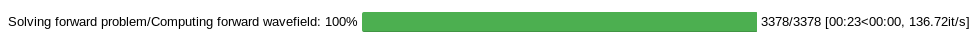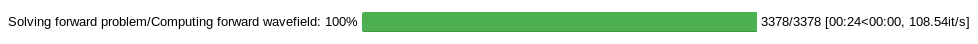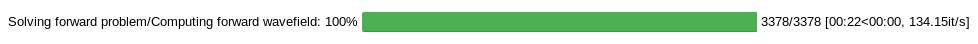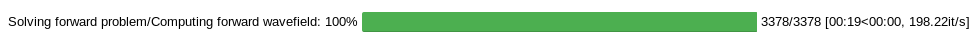Version:

This documentation is not for the latest stable Salvus version.This tutorial is presented as Python code running inside a Jupyter Notebook, the recommended way to use Salvus. To run it yourself you can copy/type each individual cell or directly download the full notebook, including all required files.This integration test compares seismograms in a 2D elastic domain with an ocean layer on top for three different scenarios:
1. The ocean layer is completely ignored and replaced by a free surface
2. The ocean layer is approximated by a load applied to the surface of the elastic domain
3. The ocean is modelled as an acoustic medium in a coupled solid/fluid simulation.
Depending on the height (weight) of the water column, we expect the solutions of  and  to be similar for (very) thin ocean layers. Furthermore, the solutions to  and  are similar, if the height of the water column corresponds to a fraction of a wavelength (here: 10%).
The ocean load is modelled as a boundary condition, which is readily available from simple_config. There are two required steps to run a simulation with ocean loading:
• Attach a scalar field OCEANLOAD to the mesh, which contains the weight of the water column, i.e., the ocean height times its density. The field is only required (and read) at the ocean surface. However, we currently don't have the concept of material properties at interface, so it still needs to have the same size as all other material parameters.
.css-fd3q3g{border-width:0px!important;padding:0px!important;font-size:85%!important;box-shadow:1px 1px 3px 1px rgba(0,0,0,0.48)!important;}import salvus.flow.simple_config as sc
sim = sc.simulation.Waveform(mesh=mesh)
sim.add_boundary_conditions(oceanload)
Copy
%matplotlib inline
%config Completer.use_jedi = False
import os
import toml
import numpy as np
import matplotlib.pyplot as plt
import salvus.flow.api
from pyasdf import ASDFDataSet
from salvus.mesh.simple_mesh import basic_mesh
import salvus.flow.simple_config as sc

SALVUS_FLOW_SITE_NAME = os.environ.get("SITE_NAME", "local")
RANKS_PER_JOB = 2
# Domain setup (m).
max_x = 2500.0  # Distance in meters.
max_y = 700.0  # Distance in meters.
max_frequency = 40.0  # Frequency in Hz.

vp_fluid = 1500.0
rho_fluid = 1200.0
def generate_meshes(ocean_height):
"NAME         ocean_benchmark\n",
"UNITS        km\n",
]

solid_model = [
"    0.7   2.6     1.7     1.0\n",
"    0.0   7.6     5.1     3.0\n",
]

ocean_layer = [
f"    {1 + ocean_height/1000} {rho_fluid/1000}     {vp_fluid/1000}     0.0\n"
f"    0.7   {rho_fluid/1000}     {vp_fluid/1000}     0.0\n"
]

with open("solid.bm", "w") as f:
f.writelines(solid_model)

with open("coupled.bm", "w") as f:
f.writelines(ocean_layer)
f.writelines(solid_model)

mesh = basic_mesh.CartesianFromBm2D(
bm_file="solid.bm",
x_max=max_x,
y_max=max_y,
max_frequency=max_frequency,
).create_mesh()
mesh_coupled = basic_mesh.CartesianFromBm2D(
bm_file="coupled.bm",
x_max=max_x,
y_max=max_y + ocean_height,
max_frequency=max_frequency,
).create_mesh()

mesh.attach_field(
ocean_height
* rho_fluid
* np.ones_like(mesh.element_nodal_fields["VP"]),
)

return mesh, mesh_coupled
# Sources.
fx, fy = 1e9, -1e10
sx, sy = 500.0, 500.0
stf = sc.source.stf.Ricker(center_frequency=14.5)
source = sc.source.cartesian.VectorPoint2D(
x=sx, y=sy, fx=fx, fy=fy, source_time_function=stf
)

nr = 10  # Number of receivers.
ry0 = 690.0  # Receiver y-value.
rx0 = 1500.0  # x-value of first receiver.
rx1 = 2000.0  # x-value of last receiver.
x=x, y=ry0, station_code=f"{_i:03d}", fields=["displacement"]
)
for _i, x in enumerate(np.linspace(rx0, rx1, nr))
]
def get_simulation_template(mesh, output=False):
sim = sc.simulation.Waveform(
)

sim.physics.wave_equation.end_time_in_seconds = 0.75

if output:
sim.output.volume_data.filename = "wavefield.h5"
sim.output.volume_data.format = "hdf5"
sim.output.volume_data.fields = ["displacement"]
sim.output.volume_data.sampling_interval_in_time_steps = 50

boundaries = sc.boundary.Absorbing(
side_sets=["x0", "x1", "y0"], taper_amplitude=0.0, width_in_meters=0.0
)
sim.validate()

return sim
def compare_seismograms(asdf_file, asdf_reference, freqmax, freqmin):
with ASDFDataSet(asdf_file, mode="r") as data, ASDFDataSet(
asdf_reference, mode="r"
) as ref:
total_misfit = 0
for u, uref in zip(data.waveforms, ref.waveforms):
tr_misfit = []
for j, cmp in enumerate(["X", "Y"]):
dt = 1e-4
tr = u.displacement.select(component=cmp)
tr.interpolate(method="lanczos", a=12, sampling_rate=1 / dt)
tr1 = tr.copy().filter(
"bandpass",
freqmax=freqmax,
freqmin=freqmin,
corners=8,
zerophase=True,
)
tr = uref.displacement.select(component=cmp)
tr.interpolate(method="lanczos", a=12, sampling_rate=1 / dt)
tr2 = tr.copy().filter(
"bandpass",
freqmax=freqmax,
freqmin=freqmin,
corners=8,
zerophase=True,
)

diff = (tr1.data - tr2.data) ** 2
tr_misfit.append(np.sqrt(diff.sum() / (tr2.data**2).sum()))
total_misfit += (tr_misfit + tr_misfit) / 2
avg_misfit = total_misfit / len(data.waveforms)

return avg_misfit

## Compare ocean load to coupled simulation

ocean_height = 3.75

mesh, mesh_coupled = generate_meshes(ocean_height)
sim = get_simulation_template(mesh)

sim_ocean = sim.copy()
sim_ocean.validate()

sim_coupled = sim.copy()
sim_coupled.set_mesh(mesh_coupled)
boundaries = sc.boundary.HomogeneousDirichlet(side_sets=["y1"])
sim_coupled.validate()

salvus.flow.api.run(
site_name=SALVUS_FLOW_SITE_NAME,
input_file=sim_ocean,
ranks=RANKS_PER_JOB,
output_folder="ocean",
get_all=True,
overwrite=True,
)
salvus.flow.api.run(
site_name=SALVUS_FLOW_SITE_NAME,
input_file=sim_coupled,
ranks=RANKS_PER_JOB,
output_folder="coupled",
get_all=True,
overwrite=True,
)

chi = compare_seismograms(
)
print("average misfit between ocean load and coupled simulation: ", chi)
assert chi < 0.01
SalvusJob job_2309101547438655_d16fba6202 running on local with 2 rank(s).
Site information:
* Salvus version: 0.12.13
* Floating point size: 32* Downloaded 310.6 KB of results to ocean.
* Total run time: 24.67 seconds.
* Pure simulation time: 24.13 seconds.
SalvusJob job_2309101547076971_8cd0e0a76b running on local with 2 rank(s).
Site information:
* Salvus version: 0.12.13
* Floating point size: 32* Downloaded 310.6 KB of results to coupled.
* Total run time: 25.51 seconds.
* Pure simulation time: 24.97 seconds.
average misfit between ocean load and coupled simulation:  0.002808968149419275


## Compare ocean load to free surface

Note that the average misfit here is on the same order of magnitude as in the previous simulation despite the fact that the ocean height is only 0.01 instead of 3.75
ocean_height = 0.01

mesh, mesh_coupled = generate_meshes(ocean_height)
sim = get_simulation_template(mesh)

sim_ocean = sim.copy()
sim_ocean.validate()

salvus.flow.api.run(
site_name=SALVUS_FLOW_SITE_NAME,
input_file=sim,
ranks=RANKS_PER_JOB,
output_folder="free_surface",
get_all=True,
overwrite=True,
)
salvus.flow.api.run(
site_name=SALVUS_FLOW_SITE_NAME,
input_file=sim_ocean,
ranks=RANKS_PER_JOB,
output_folder="ocean",
get_all=True,
overwrite=True,
)
chi = compare_seismograms(
)
print("average misfit between free surface and coupled simulation: ", chi)
assert chi < 0.01
SalvusJob job_2309101548747270_469ba22044 running on local with 2 rank(s).
Site information:
* Salvus version: 0.12.13
* Floating point size: 32* Downloaded 310.5 KB of results to free_surface.
* Total run time: 23.35 seconds.
* Pure simulation time: 22.80 seconds.
SalvusJob job_2309101548098461_232a773e04 running on local with 2 rank(s).
Site information:
* Salvus version: 0.12.13
* Floating point size: 32* Downloaded 310.6 KB of results to ocean.
* Total run time: 21.11 seconds.
* Pure simulation time: 20.81 seconds.
average misfit between free surface and coupled simulation:  0.0004279289121761561PAGE CONTENTS